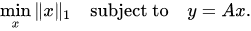# Solver status is Infeasible

(Prateek Nallabolu) #1

Hello,
I am trying to solve a basis pursuit algorithm (Compressed sensing) using CVX. The algorithm is given as:In my case, “A” is the multiplication of two matrices “Directivity” and “Baseband”. Since each element of the matrix “baseband” is also a complex 1-D array (1x1000), I couldn’t set up the algorithm directly in CVX. I used the “expression” feature in CVX to manually calculate the product “Ax”. The matrix “y” is of the size 10x1000.

``````cvx_begin
variable x(399)
expression A(10,1000)
for ii=1:10
for jj=1:19
for kk=1:21
A(ii,:)= A(ii,:)+ x((jj-1)*21+kk).*directivity(ii,kk).*baseband(jj,:);
end
end
end
minimize(norm(x,1))
subject to
y == A
cvx_end
``````

## Calling SDPT3 4.0: 20798 variables, 798 equality constraints For improved efficiency, SDPT3 is solving the dual problem.

num. of constraints = 798
dim. of socp var = 798, num. of socp blk = 399
dim. of free var = 20000 *** convert ublk to lblk

SDPT3: Infeasible path-following algorithms

## number of iterations = 31 residual of dual infeasibility certificate X = 1.50e-10 reldist to infeas. <= 2.82e-12 Total CPU time (secs) = 483.76 CPU time per iteration = 15.61 termination code = 2 DIMACS: 9.5e+00 0.0e+00 9.0e+02 0.0e+00 -1.0e+00 8.7e+00

Status: Infeasible
Optimal value (cvx_optval): +Inf

The status of the solver is Infeasible. I am not sure if I am using the “expression” feature correctly. I went through the CVX user guide but I couldn’t figure out the error. Can anyone please point out the error in the code?
I am not very familiar with CVX and any help will be greatly appreciated.

(Mark L. Stone) #2

I don’t understand what you have done, and can’t say whether it is correct, but it looks rather dubious to me. Why is `x` 399 by 1 rather than 1000 by 1?

Perhaps A could be a 3-D array, 10 by 1000 by 1000. Then you could specify the following as the constraints in CVX?

``````for i=1:1000
y(:,i) == A(:,:,i)*x
end
``````

Does that specify what you want, presuming you construct `A` correctly?

Anyhow, i don’t know much about compressed sensing, but the constraint(s) y == Ax (for instance per my for loop above) seems like it would not be the “correct” constraint to have, and would indeed be overconstrained such that it would be almost impossible to be feasible. Do you perhaps want a norm constraint on y-Ax or as a term in the objective function?

(Prateek Nallabolu) #3

Thank you for the suggestion of using a for loop in the constraints and making “A” 3-D array. I’ve restructured the CVX program without using expressions and used a for loop in the constraints. I am able to solve the algorithm now.

A = zeros(1000,399,10);
for ii=1:10
for jj=1:1000
for kk=1:399
col = uint8(mod(kk-1,21));
col1 = uint8(floor((kk-1)/21));
A(jj,kk,ii) = directivity(ii,col+1)*baseband(col1+1,jj);
end
end
end
cvx_begin
variable x(399,1)
minimize(norm(x,1))
subject to
for ii=1:10
for jj=1:1000
y(ii,jj) == A(jj,:,ii)*x
end
end
cvx_end

Sorry for not being clear with my problem earlier. I’ve tried to explain my constraint in the image below. Hope it explains why x is 399 by 1 and not 1000 by 1.

The equality constraint y=Ax works because I am considering a noiseless environment. In the case of noise, as you mentioned I will have a constraint on the L2 norm of y-Ax.
Thank you once again for your help.

(Mark L. Stone) #4

For the record, I corrected `y(i,:)` to `y(:,i)` in my previous post.

(Prateek Nallabolu) #5

The first approach using “expression” also works. I made a small mistake in the MATLAB program.
Thank you once again for your support.